# Exploratory analysis

Data Visualization, part 1. Code for quiz 7.

1. Load the R package that we will use.
``````library(tidyverse)
``````

# Question: Modify Slide 34

• Create a plot with `faithful` dataset.

• Add points with `geom_point`

• Assign the variable `eruptions` to the x-axis

• Assign the variable `waiting` to the y-axis

• Colour the points according to whether `waiting` is smaller or greater than 60.

``````ggplot(faithful) +
geom_point(aes(x = eruptions, y = waiting, colour = eruptions < 60))
``````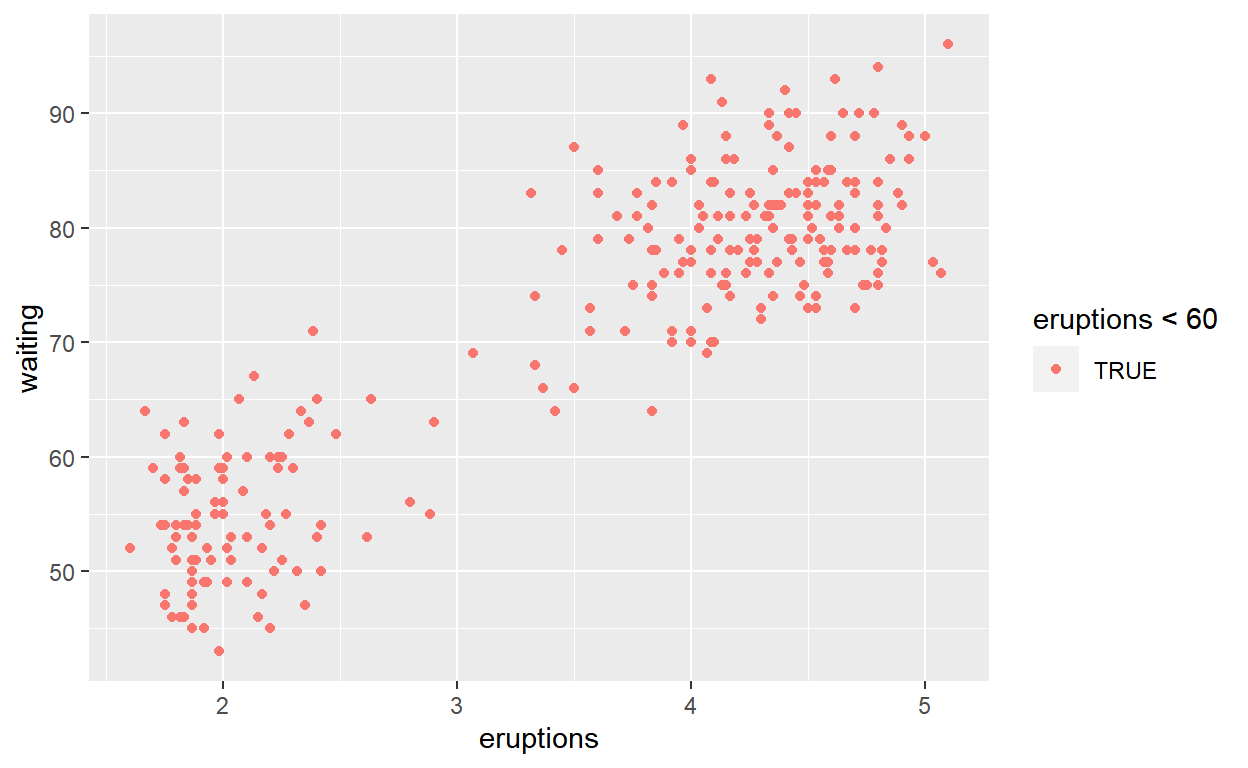# Question: Modify Slide 35

• Create a plot with `faithful` dataset

• Add points with `geom_point`

• Assign the variable `eruptions` to the x-axis

• Assign the variable `waiting` to the y-axis

• Assign the color `dodgerblue` to all points

``````ggplot(faithful) +
geom_point(aes(x = eruptions, y = waiting),
colour = 'dodgerblue')
``````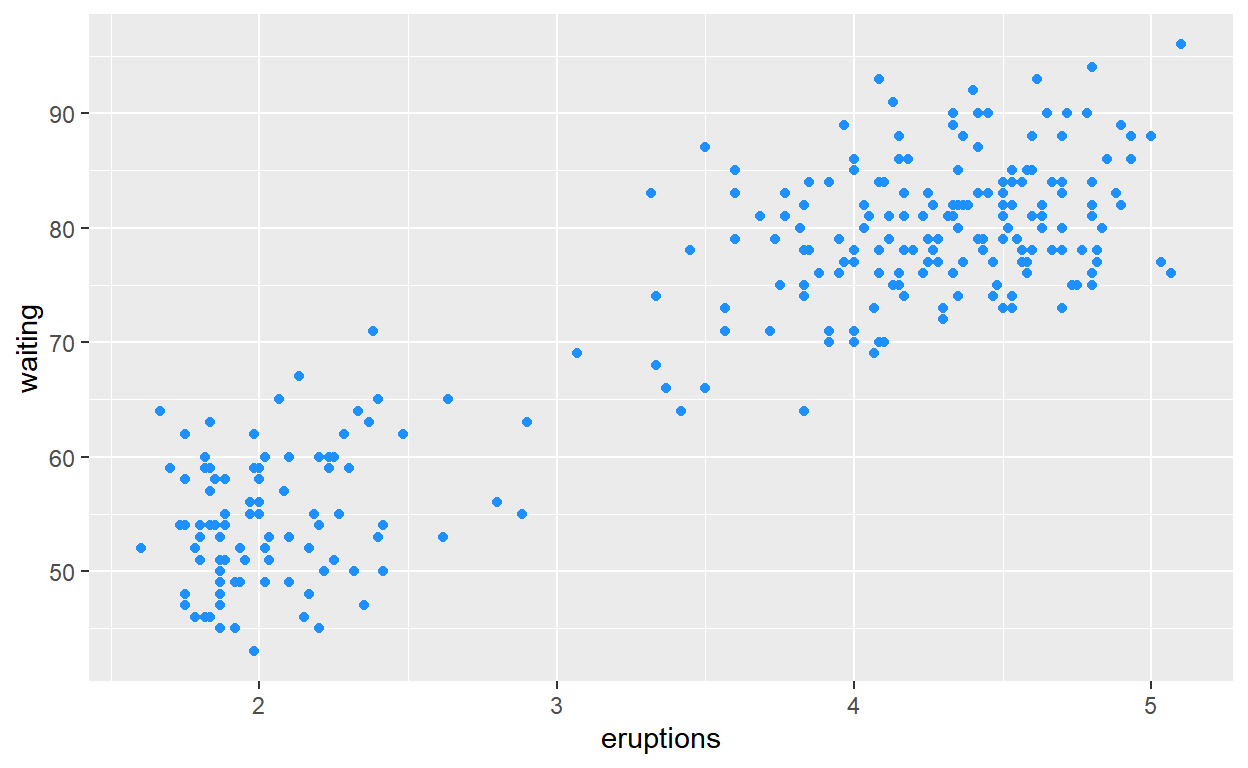# Question: Modify Slide 36

• Create a plot with `faithful` dataset

• Use `geom_histogram()` to plot the distribution of `waiting` time

• Assign the variable `waiting` to the x-axis
``````ggplot(faithful) +
geom_histogram(aes(x = waiting))
``````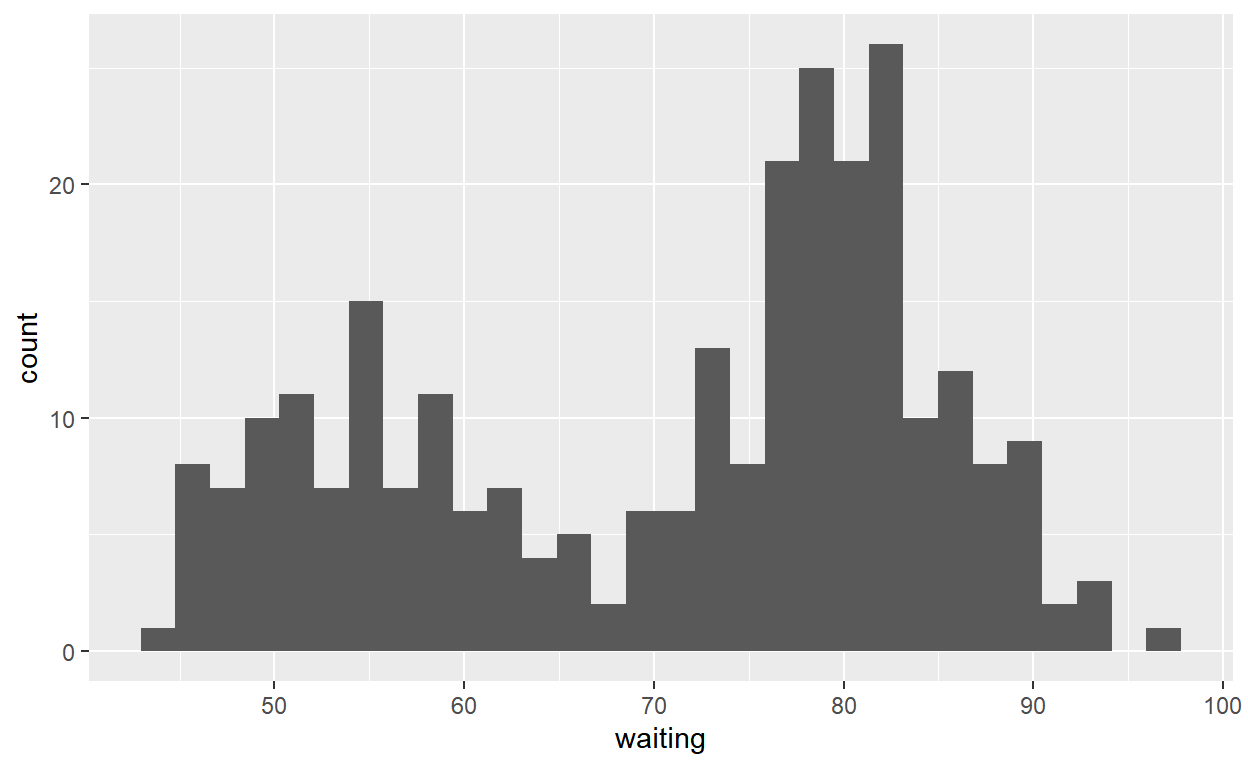# Question: Modify geom-ex-1

• Create a plot with the `faithful` dataset

• Add points with `geom_point`

• Assign the variable `eruptions` to the x-axis

• Assign the variable `waiting` to the y-axis

• Set the shape of the points to `Square`

• Set the point size to `5`

• Set the point transparency `0.5`

``````ggplot(faithful) +
geom_point(aes(x = eruptions, y = waiting),
shape = "square", size = 5, alpha = 0.5)
``````# Question: Modify geom-ex-2

• Create a plot with `faithful` dataset

• Use `geom_histogram()` to plot distribution of the `eruptions` (time)

• Fill in the histogram based on whether eruptions are greater than or less than 3.2

``````ggplot(faithful) +
geom_histogram(aes(x = eruptions, fill = eruptions > 3.2))
``````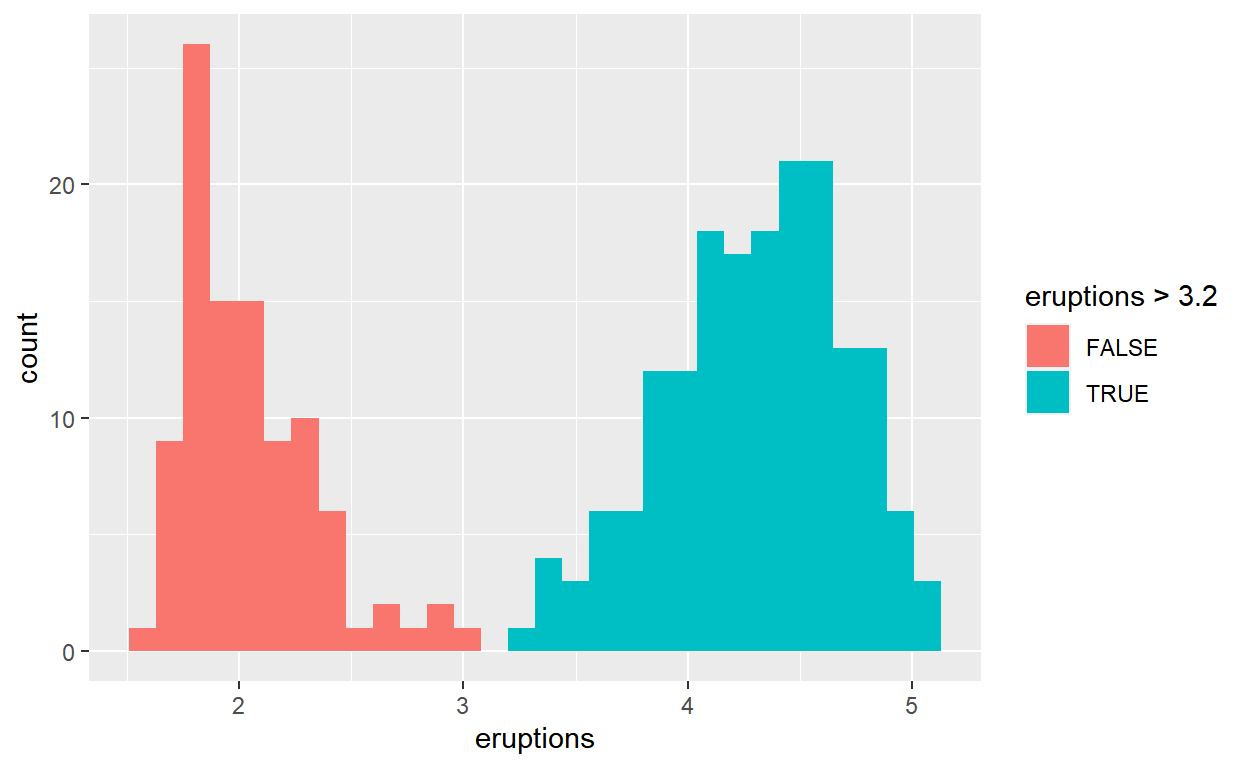# Question: Modify stat-slide-40

• Create plot with `mpg` dataset

• Add `geom_bar()` to create a bar chart of the variable `manufacturer`

``````ggplot(mpg) +
geom_bar(aes(x = manufacturer))
``````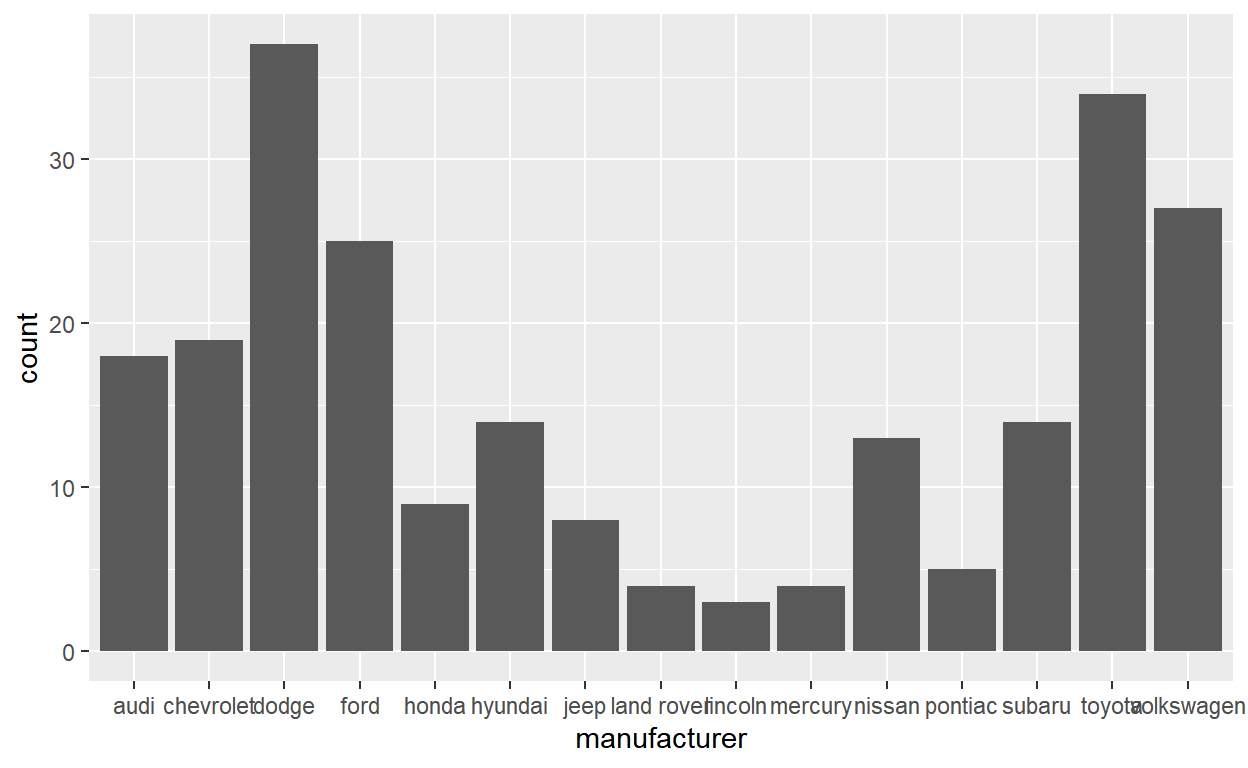# Question: Modify stat-slide-41

• Change code count and plot the variable `manufacturer` instead of class.
``````mpg_counted <- mpg %>%
count(manufacturer, name = 'count')
ggplot(mpg_counted) +
geom_bar(aes(x = manufacturer, y = count), stat = 'identity')
``````# Question: Modify stat-slide-43

• Change code to plot bar chart of each manufacturer as a percent of total

• Change `class` to `manufacturer`

``````ggplot(mpg) +
geom_bar(aes(x = manufacturer, y = after_stat(100 * count / sum(count))))
``````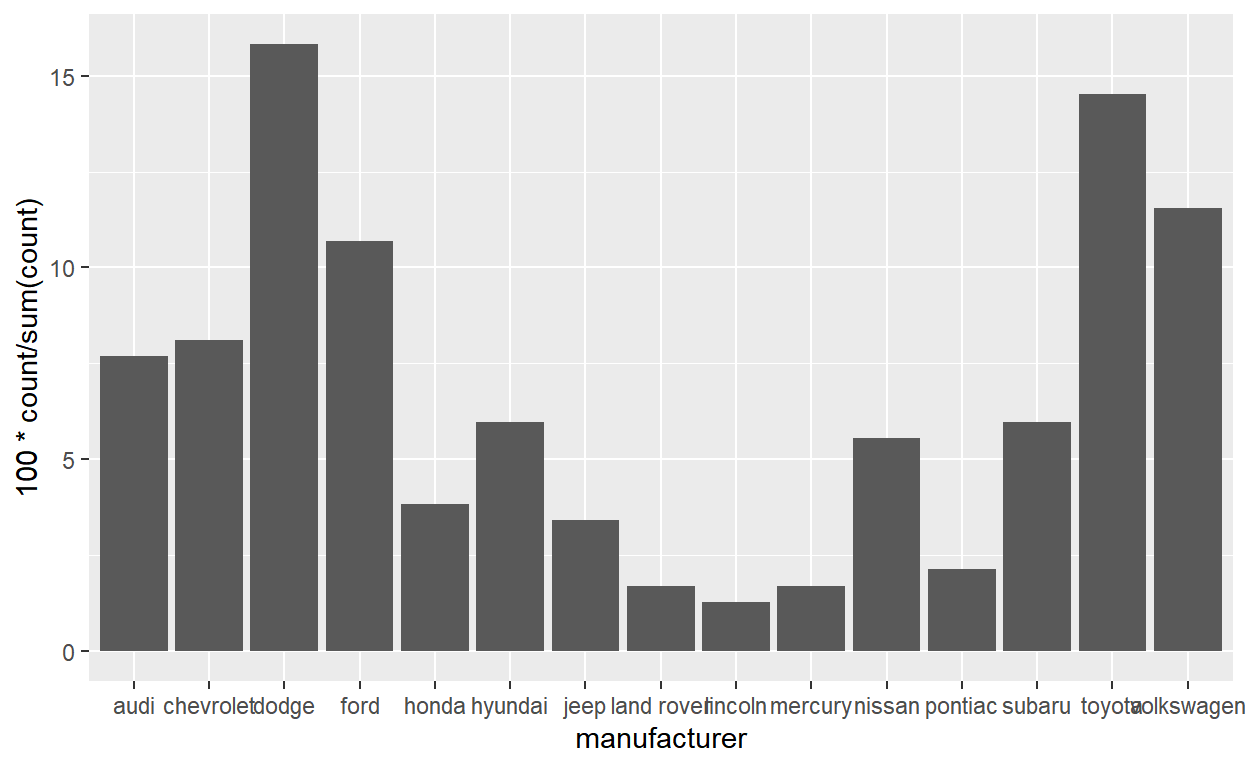# Question: Modify stat-ex-2

• Use `stat_summary()` to add a dot at the `median` of each group

• Color the dot `dodgerblue`

• Make the shape of the dot `plus`

• Make the dot size `2`

``````ggplot(mpg) +
geom_jitter(aes(x = class, y = hwy), width = 0.2) +
stat_summary(aes(x = class, y = hwy), geom = "point",
fun = "median", color = "dodgerblue", shape = "plus", size = 2)
``````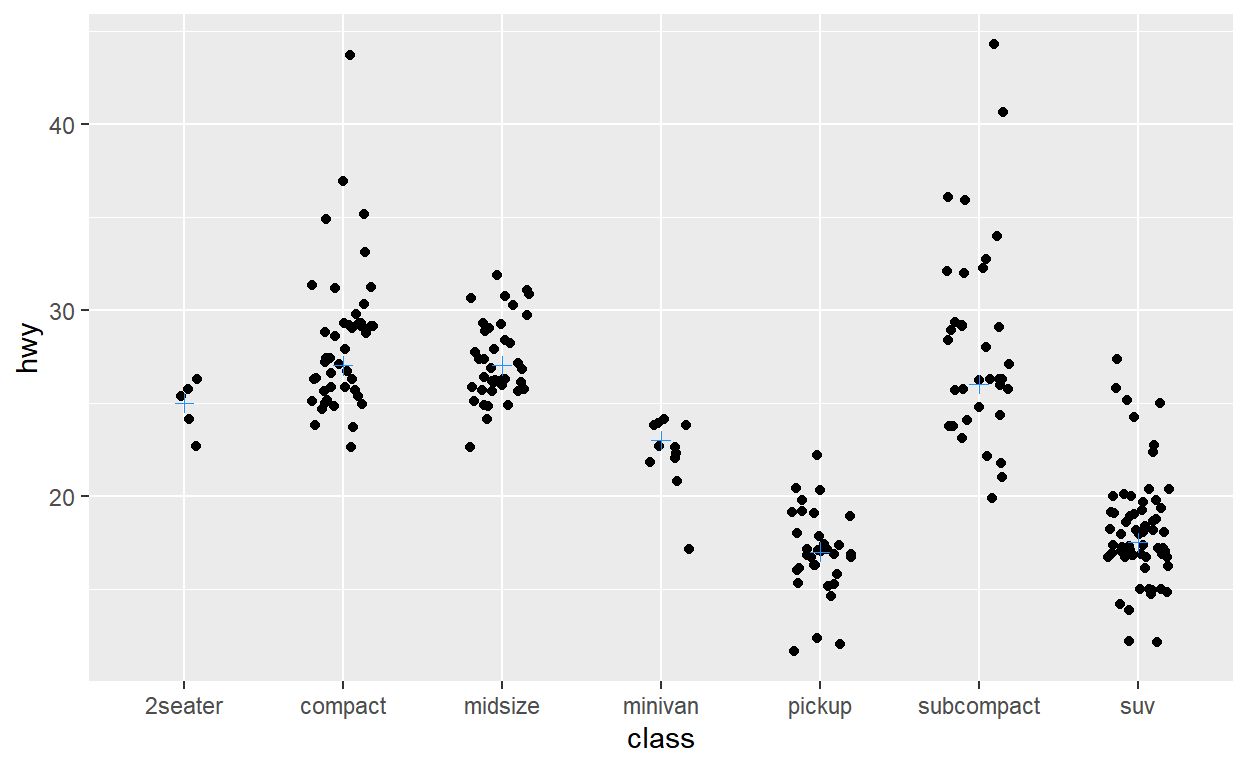``````ggsave(filename = "preview.png",
path = here::here("_posts", "2022-03-11-exploratory-analysis"))
``````